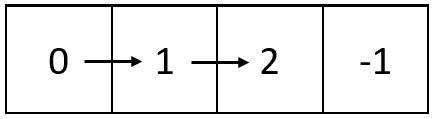##### Welcome to Subscribe On Youtube

Formatted question description: https://leetcode.ca/all/2326.html

# 2326. Spiral Matrix IV

• Difficulty: Medium.
• Related Topics: Array, Linked List, Matrix, Simulation.
• Similar Questions: Spiral Matrix, Spiral Matrix II, Spiral Matrix III.

## Problem

You are given two integers m and n, which represent the dimensions of a matrix.

You are also given the head of a linked list of integers.

Generate an m x n matrix that contains the integers in the linked list presented in spiral order (clockwise), starting from the top-left of the matrix. If there are remaining empty spaces, fill them with -1.

Return the generated matrix.

Example 1:Input: m = 3, n = 5, head = [3,0,2,6,8,1,7,9,4,2,5,5,0]
Output: [[3,0,2,6,8],[5,0,-1,-1,1],[5,2,4,9,7]]
Explanation: The diagram above shows how the values are printed in the matrix.
Note that the remaining spaces in the matrix are filled with -1.


Example 2:Input: m = 1, n = 4, head = [0,1,2]
Output: [[0,1,2,-1]]
Explanation: The diagram above shows how the values are printed from left to right in the matrix.
The last space in the matrix is set to -1.


Constraints:

• 1 <= m, n <= 105

• 1 <= m * n <= 105

• The number of nodes in the list is in the range [1, m * n].

• 0 <= Node.val <= 1000

## Solution (Java, C++, Python)

• /**
* public class ListNode {
*     int val;
*     ListNode next;
*     ListNode() {}
*     ListNode(int val) { this.val = val; }
*     ListNode(int val, ListNode next) { this.val = val; this.next = next; }
* }
*/
class Solution {
private enum Direction {
RIGHT,
DOWN,
LEFT,
UP
}

public int[][] spiralMatrix(int m, int n, ListNode head) {
int[][] arr = new int[m][n];
int i = 0;
int j = -1;
Direction direction = Direction.RIGHT;
// Boundaries
// ++ after Left to right Horizontal traversed
int a = 0;
// -- after Down to Up vertical traversed
int b = n - 1;
// -- after Right to Left horizontal teversed
int c = m - 1;
// ++ after Down to Up vertical traversed
int d = 0;
for (int k = 0; k < m * n; ++k) {
int val = -1;
}
switch (direction) {
case RIGHT:
++j;
if (j == b) {
direction = Direction.DOWN;
++a;
}
break;
case DOWN:
++i;
if (i == c) {
direction = Direction.LEFT;
}
break;
case LEFT:
--j;
if (j == d) {
--c;
direction = Direction.UP;
}
break;
case UP:
default:
--i;
if (i == a) {
--b;
++d;
direction = Direction.RIGHT;
}
break;
}
arr[i][j] = val;
}
return arr;
}
}

• Todo

• # Definition for singly-linked list.
# class ListNode:
#     def __init__(self, val=0, next=None):
#         self.val = val
#         self.next = next
class Solution:
def spiralMatrix(self, m: int, n: int, head: Optional[ListNode]) -> List[List[int]]:
ans = [[-1] * n for _ in range(m)]
i = j = p = 0
dirs = [[0, 1], [1, 0], [0, -1], [-1, 0]]
while 1:
break
while 1:
x, y = i + dirs[p], j + dirs[p]
if x < 0 or y < 0 or x >= m or y >= n or ~ans[x][y]:
p = (p + 1) % 4
else:
i, j = x, y
break
return ans

############

# 2326. Spiral Matrix IV
# https://leetcode.com/problems/spiral-matrix-iv/

# class ListNode:
#     def __init__(self, val=0, next=None):
#         self.val = val
#         self.next = next
class Solution:
def spiralMatrix(self, rows: int, cols: int, head: Optional[ListNode]) -> List[List[int]]:
matrix = [[-1] * cols for _ in range(rows)]

rowStart, rowEnd = 0, rows - 1
colStart, colEnd = 0, cols - 1

# traverse right
i = colStart
while head and i <= colEnd:
i += 1
rowStart += 1

# traverse down
i = rowStart
while head and i <= rowEnd:
i += 1
colEnd -= 1

# traverse left
i = colEnd
while head and i >= colStart:
i -= 1
rowEnd -= 1

# traverse up
i = rowEnd
while head and i >= rowStart:
i -= 1
colStart += 1

return matrix



Explain:

nope.

Complexity:

• Time complexity : O(n).
• Space complexity : O(n).